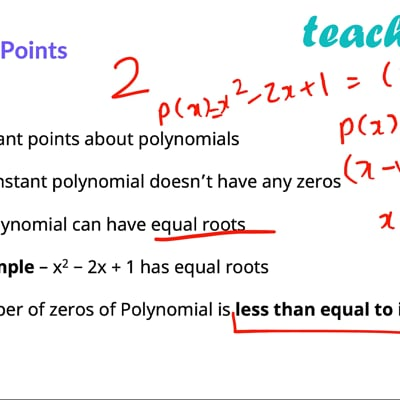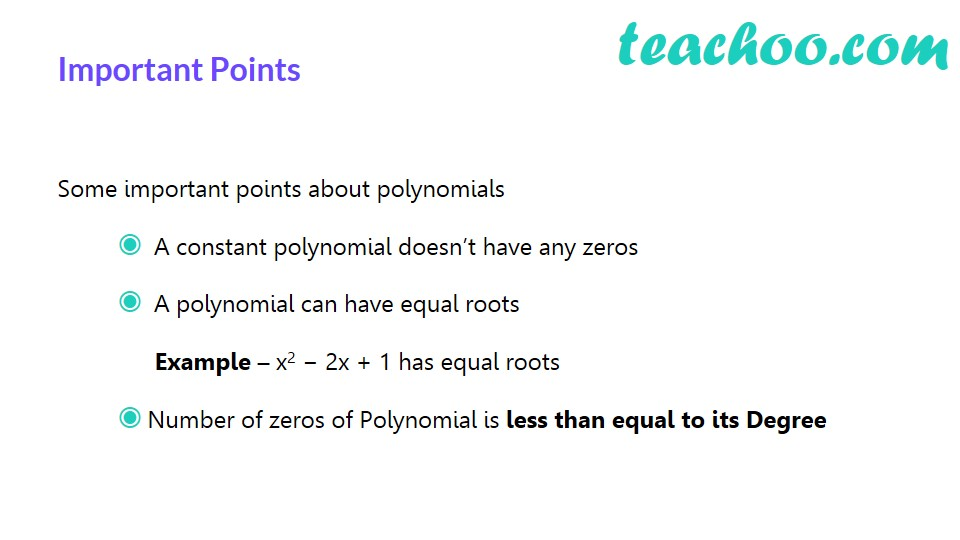Finding Zeroes of a polynomial

Chapter 2 Class 9 Polynomials
Concept wiseThis video is only available for Teachoo black usersThis video is only available for Teachoo black usersThis video is only available for Teachoo black usersIf  p(a) = 0

Then x = a is the zero of polynomial

So, to find zero,

We put p(x) = 0

And then find the value of x

Example :

p(x) = x + 30

Putting p(x) = 0

x + 30 = 0

x =   –30

So, x = −30 is a zero of polynomial p(x)

Solve all your doubts with Teachoo Black (new monthly pack available now!)

### Transcript

How to find zeroes of polynomial? For polynomial p(x) , If p(a) = 0 Then x = a is the zero of polynomial So, to find zero, We put p(x) = 0 And then find the value of x An Example Find zero of polynomial p(x) = x + 30 Given p(x) = x + 30 Putting p(x) = 0 x + 30 = 0 x = −30 So, x = −30 is a zero of polynomial p(x) One more… Find zeros of polynomial p(x) = x2 − 9 Given p(x) = x2 −9 Putting p(x) = 0 x2 − 9 = 0 x2 = 9 x = ±3 So, x = 3, −3 are the zeros of polynomial p(x) Important Points Important Points Some important points about polynomials A constant polynomial doesn’t have any zeros A polynomial can have equal roots Example – x2 − 2x + 1 has equal roots Number of zeros of Polynomial is less than equal to its Degree